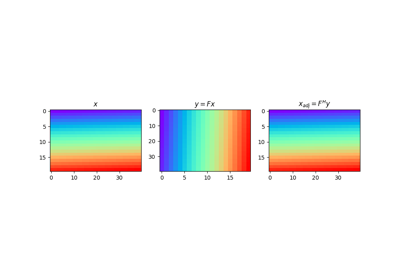# pylops.Transpose¶

class pylops.Transpose(dims, axes, dtype='float64')[source]

Transpose operator.

Transpose axes of a multi-dimensional array. This operator works with flattened input model (or data), which are however multi-dimensional in nature and will be reshaped and treated as such in both forward and adjoint modes.

Parameters: dims : tuple, optional Number of samples for each dimension (None if only one dimension is available) axes : tuple, optional Direction along which symmetrization is applied dtype : str, optional Type of elements in input array ValueError If axes contains repeated dimensions (or a dimension is missing)

Notes

The Transpose operator reshapes the input model into a multi-dimensional array of size dims and transposes (or swaps) its axes as defined in axes.

Similarly, in adjoint mode the data is reshaped into a multi-dimensional array whose size is a permuted version of dims defined by axes. The array is then rearragned into the original model dimensions dims.

Attributes: shape : tuple Operator shape explicit : bool Operator contains a matrix that can be solved explicitly (True) or not (False)

Methods

 __init__(self, dims, axes[, dtype]) Initialize this LinearOperator. adjoint(self) Hermitian adjoint. cond(self, \*\*kwargs_eig) Condition number of linear operator. conj(self) Complex conjugate operator div(self, y[, niter]) Solve the linear problem $$\mathbf{y}=\mathbf{A}\mathbf{x}$$. dot(self, x) Matrix-matrix or matrix-vector multiplication. eigs(self[, neigs, symmetric, niter]) Most significant eigenvalues of linear operator. matmat(self, X) Matrix-matrix multiplication. matvec(self, x) Matrix-vector multiplication. rmatvec(self, x) Adjoint matrix-vector multiplication. transpose(self) Transpose this linear operator.

## Examples using pylops.Transpose¶Transpose